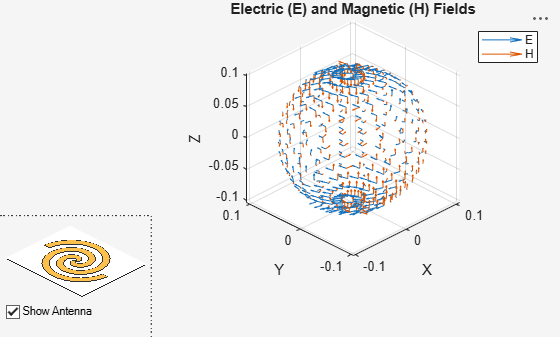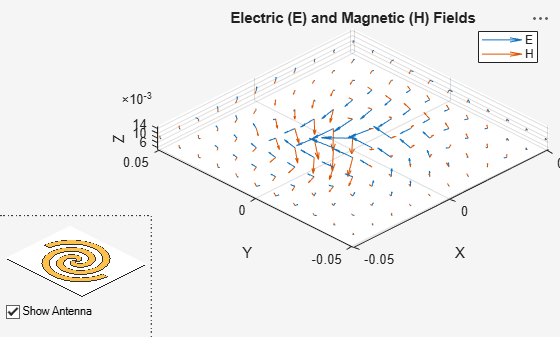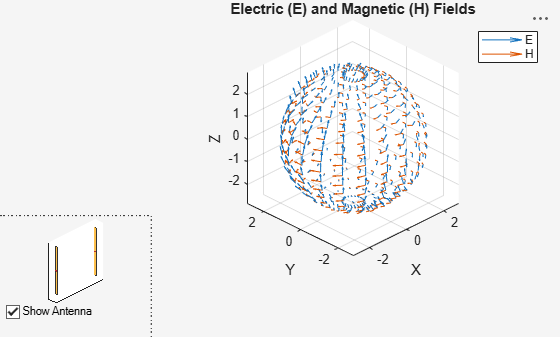EHfields

Electric and magnetic fields of antennas; Embedded electric and magnetic fields of antenna element in arrays

Description

[e,h] = EHfields(object,frequency) calculates the x, y, and z components of electric field and magnetic field of an antenna or array object at a specified frequency.

example

EHfields(object,frequency) plots the absolute values of the electric and magnetic field vectors along with corresponding signed complex angles at the specified frequency values and at the specified points in space. The multiplication factor with absolute field value is +1 for positive and -1 for negative complex angle.

example

[e,h] = EHfields(object,frequency,points) calculates the x, y, and z components of electric field and magnetic field of an antenna or array object. These fields are calculated at specified points in space and at a specified frequency.

example

EHfields(object, frequency, points) plots the absolute values of the electric and magnetic field vectors along with corresponding signed complex angles at specified frequency values and at specified points in space. The multiplication factor with absolute field value is +1 for positive and -1 for negative complex angle.

EHfields(___,Name,Value) plots the electric and magnetic field vectors using one or more name-value arguments in addition to any of the input argument combinations in previous syntaxes. For example, 'ViewField','E' displays only the electric field.

Use the 'ElementNumber' and 'Termination' properties to calculate the embedded electric and magnetic fields of the antenna element in an array connected to a voltage source. The voltage source model consists of an ideal voltage source of 1 volt in series with a source impedance. The embedded pattern includes the effect of mutual coupling due to the other antenna elements in the array.

Examples

collapse all

Plot electric and magnetic fields of a default Archimedean spiral antenna.

h = spiralArchimedean;
EHfields(h,4e9)Calculate electric and magnetic fields at a point 1m along the z-axis from an Archimedean spiral antenna.

h = spiralArchimedean;
[e,h] = EHfields(h,4e9,[0;0;1])
e = 3×1 complex

-0.4145 - 0.2545i
-0.3036 + 0.4095i
0.0000 - 0.0000i

h = 3×1 complex

0.0008 - 0.0011i
-0.0011 - 0.0007i
-0.0000 + 0.0000i

Calculate electric and magnetic fields at a point 1 m along the z-axis from an Archimedean spiral antenna. Return the field as azimuth, elevation, and radius values in a spherical coordinate system.

h = spiralArchimedean;
[e,h] = EHfields(h,4e9,[0;0;1],'CoordinateSystem','spherical')
e = 3×1 complex

-0.3036 + 0.4095i
0.4145 + 0.2545i
0.0000 - 0.0000i

h = 3×1 complex

-0.0011 - 0.0007i
-0.0008 + 0.0011i
-0.0000 + 0.0000i

Create an Archimedean spiral antenna. Plot electric and magnetic field vector at the z = 1cm plane from the antenna.

h = spiralArchimedean;

Define points on a rectangular grid in the X-Y plane.

[X,Y] = meshgrid(-.05:.01:.05,-.05:.01:.05);

p = [X(:)';Y(:)';.01*ones(1,prod(size(X)))];

Plot electric and magnetic field vector at the z = 1cm plane. from the antenna

EHfields (h,4e9,p)Plot the embedded vector fields of a linear array when the first element is excited and all the other antenna elements are terminated using 50-ohm resistance.

l = linearArray;
EHfields(l, 70e6, 'ElementNumber', 1, 'Termination', 50);Calculate electric and magnetic fields of a dipole antenna.

[E H] = EHfields(dipole, 70e6:2e6:80e6, [0 0 0]')
E = 3×6 complex

0.0000 - 0.0000i   0.0000 - 0.0000i   0.0000 - 0.0000i   0.0000 - 0.0000i   0.0000 - 0.0000i   0.0000 - 0.0000i
-0.0242 + 0.0030i  -0.0251 + 0.0028i  -0.0263 + 0.0025i  -0.0277 + 0.0023i  -0.0292 + 0.0021i  -0.0308 + 0.0020i
-1.5494 - 1.9676i  -1.8677 - 1.6821i  -2.0155 - 1.3838i  -2.0573 - 1.1327i  -2.0447 - 0.9371i  -2.0075 - 0.7881i

H = 3×6 complex
10-3 ×

-0.6066 + 0.0834i  -0.5235 + 0.1859i  -0.4344 + 0.2367i  -0.3583 + 0.2546i  -0.2985 + 0.2554i  -0.2525 + 0.2482i
0.0000 - 0.0000i   0.0000 - 0.0000i   0.0000 - 0.0000i   0.0000 - 0.0000i   0.0000 - 0.0000i   0.0000 - 0.0000i
0.0000 + 0.0000i   0.0000 + 0.0000i   0.0000 + 0.0000i   0.0000 + 0.0000i   0.0000 + 0.0000i   0.0000 + 0.0000i

Calculate the electric and magnetic fields of a helix antenna with a vertically polarized electric field.

EHfields(helix,1e9,'Polarization','V')
ans = 1×441 complex

0.0251 - 0.0286i  -0.0313 + 0.0450i  -0.0387 + 0.0590i  -0.0482 + 0.0704i  -0.0601 + 0.0786i  -0.0750 + 0.0830i  -0.0929 + 0.0828i  -0.1138 + 0.0767i  -0.1364 + 0.0634i  -0.1590 + 0.0417i  -0.1785 + 0.0110i  -0.1911 - 0.0276i  -0.1930 - 0.0713i  -0.1815 - 0.1153i  -0.1562 - 0.1537i  -0.1196 - 0.1804i  -0.0769 - 0.1911i  -0.0348 - 0.1841i   0.0005 - 0.1608i   0.0255 - 0.1251i  -0.0411 + 0.0825i   0.0251 - 0.0286i   0.0098 + 0.0396i   0.0004 + 0.0559i  -0.0125 + 0.0709i  -0.0295 + 0.0835i  -0.0508 + 0.0925i  -0.0762 + 0.0961i  -0.1049 + 0.0925i  -0.1349 + 0.0802i  -0.1632 + 0.0583i  -0.1860 + 0.0272i  -0.1991 - 0.0107i  -0.1994 - 0.0511i  -0.1852 - 0.0879i  -0.1579 - 0.1150i  -0.1217 - 0.1270i  -0.0828 - 0.1214i  -0.0478 - 0.0986i  -0.0212 - 0.0623i  -0.0039 - 0.0181i  -0.0411 + 0.0825i   0.0251 - 0.0286i   0.0494 + 0.0321i   0.0382 + 0.0508i   0.0221 + 0.0696i   0.0005 + 0.0870i  -0.0267 + 0.1007i  -0.0591 + 0.1085i  -0.0952 + 0.1080i

Input Arguments

collapse all

Antenna or array object, specified as an object.

Example: h = spiralArchimedean

Frequency used to calculate electric and magnetic fields, specified as a scalar or a vector in Hz.

Example: 70e6

Data Types: double

Cartesian coordinates of points in space, specified as a 3-by-p complex matrix. p is the number of points at which to calculate the E-H field.

Example: [0;0;1]

Data Types: double

Name-Value Arguments

Example: 'ScaleFields',[2 0.5] specifies scalar values of the electric and magnetic fields

Specify optional comma-separated pairs of Name,Value arguments. Name is the argument name and Value the corresponding value. Name must appear inside single quotes (''). You can specify several name and value pair arguments in any order as Name1,Value1,...,NameN,ValueN.

Value by which to scale the electric and magnetic fields, specified as the comma-separated pair consisting of 'ScaleFields' and a two-element vector. The first element scales the E field and the second element scales the H-field. A value of 2 doubles the relative length of either field. A value of 0.5 to halves the length of either field. A value of 0 plots either field without automatic scaling.

Example: 'ScaleFields',[2 0.5]

Data Types: double

Field to display, specified as the comma-separated pair consisting of 'ViewField' and 'E' or 'H'. 'E' displays the electric field and 'H' displays the magnetic field.

Example: 'ViewField','E'

Data Types: char

Antenna element in array, specified as the comma-separated pair consisting of 'ElementNumber' and scalar This antenna element is connected to the voltage source.

Note

Use this property to calculate the embedded pattern of an array.

Example: 'ElementNumber',1

Data Types: double

Impedance value for array element termination, specified as the comma-separated pair consisting of 'Termination' and scalar. The impedance value terminates other antenna elements of an array while calculating the embedded pattern of the antenna connected to the voltage source.

Note

Use this property to calculate the embedded pattern of an array.

Example: 'Termination',40

Data Types: double

Coordinate system to calculate and plot the electric and magnetic fields of an antenna or array, specified as the comma-separated pair consisting of 'CoordinateSystem' and 'rectangular' or 'spherical'.

When you specify both the 'CoordinateSystem' and the 'Polarization' name-value arguments, the EHfields function ignores the value of 'CoordinateSystem'. The output is the same in both coordinate systems.

Example: 'CoordinateSystem','spherical'

Data Types: char

Polarization of the electric field, specified as the comma-separated pair consisting of 'Polarization' and 'H' for horizontal polarization and 'V' for vertical polarization. The magnetic field has the opposite polarization.

When you specify 'Polarization' in a syntax without output arguments, the EHfields function returns the component of the electric field with polarization as a 1-by-p vector in the variable ans. The function does not plot the values.

Example: 'Polarization','H'

Data Types: char

Output Arguments

collapse all

x, y, z components of electrical field in the rectangular coordinate system or azimuth, elevation, radial components in the spherical coordinate system, returned as 3-by-p complex matrix in V/m. The dimension p is the number of points in space at which the electric and magnetic fields are computed.

x, y, z components of magnetic field in the rectangular coordinate system or azimuth, elevation, radial components in the spherical coordinate system, returned as a 3-by-p complex matrix in A/m. The dimension p is the number of points in space at which the electric and magnetic fields are computed.